# The periodic nature of SHM and simple pendulum#### Everything You Need in One Place

Homework problems? Exam preparation? Trying to grasp a concept or just brushing up the basics? Our extensive help & practice library have got you covered.#### Learn and Practice With Ease

Our proven video lessons ease you through problems quickly, and you get tonnes of friendly practice on questions that trip students up on tests and finals.#### Instant and Unlimited Help

Our personalized learning platform enables you to instantly find the exact walkthrough to your specific type of question. Activate unlimited help now!

##### Intros
###### Lessons
1. Periodic Motion
2. Position Vs. Time Graph
3. Velocity Vs. Time Graph
4. Acceleration Vs. Time Graph
5. Simple Pendulum
##### Examples
###### Lessons
1. The spring of a 1200kg car compresses 2.0mm when its 75kg driver gets into the car. If the car goes over a bump, what will be the frequency of the vibration?
1. A 6 kg block is attached to a spring wit a spring constant of 216 N/m. The spring is stretched to a length of 12cm and then released.
1. What is the position of the block after 2.0s?
2. What is the speed of the block after 2.0s?
3. What is the acceleration of the block after 2.0s?
2. A mass of 2.40 kg is attached to a horizontal spring with a spring constant of 121N/m. It is stretched to a length of 10.0cm and released from test.
1. Write down the equation for the position and velocity of the block as a function of time.
2. What is the maximum magnitude of acceleration that the block experiences? Is this consistent with Hook's Law?
3. A mass is attached to a horizontal spring, and oscillates with a period of 1.4s and with an amplitude of 12cm. At $t=0$s, the mass is 12cm to the right of the equilibrium positon.
1. Write down the function for the position, velocity, and acceleration of the mass as a function of time.
2. Plot the position Vs. time and Velocity Vs. time from 0s to 2.8s. On each graph label period, initial value and amplitude.
3. At what position is the speed of the block half of the maximum speed?
4. A simple Pendulum has a length of 42.0cm and makes 62.0 complete oscillation in 3.0 min.
1. Find the period of the pendulum
2. Find the value of g at the location of the pendulum.
5. The length of a simple pendulum in 0.86m, the pendulum bob has a mass of 265 g and it is released to an angle of 11.0° to the vertical.
1. What is the frequency of vibration?
2. What is the pendulum bob's speed at the lowest point?
3. What is the total energy stored in this oscillation?
###### Free to Join!
StudyPug is a learning help platform covering math and science from grade 4 all the way to second year university. Our video tutorials, unlimited practice problems, and step-by-step explanations provide you or your child with all the help you need to master concepts. On top of that, it's fun - with achievements, customizable avatars, and awards to keep you motivated.
• #### Easily See Your ProgressWe track the progress you've made on a topic so you know what you've done. From the course view you can easily see what topics have what and the progress you've made on them. Fill the rings to completely master that section or mouse over the icon to see more details.
• #### Make Use of Our Learning Aids###### Practice Accuracy

Get quick access to the topic you're currently learning.

See how well your practice sessions are going over time.

Stay on track with our daily recommendations.

• #### Earn Achievements as You LearnMake the most of your time as you use StudyPug to help you achieve your goals. Earn fun little badges the more you watch, practice, and use our service.
• #### Create and Customize Your AvatarPlay with our fun little avatar builder to create and customize your own avatar on StudyPug. Choose your face, eye colour, hair colour and style, and background. Unlock more options the more you use StudyPug.
###### Topic Notes

In this lesson, we will learn:

• The nature of the periodic motion
• The graph analysis of the periodic motion
• Simple Pendulum

Notes:

• If each vibration (the back and forth motion) takes the same amount of time, then the motion is Periodic.
• To discuss the period motion we need to define the following terms;
• Cycle; a complete to-and-fro movement.
• Amplitude; maximum displacement, the greatest distance from the equilibrium position.
• Period; time taken for one complete oscillation.
• Frequency; number of oscillations in one second.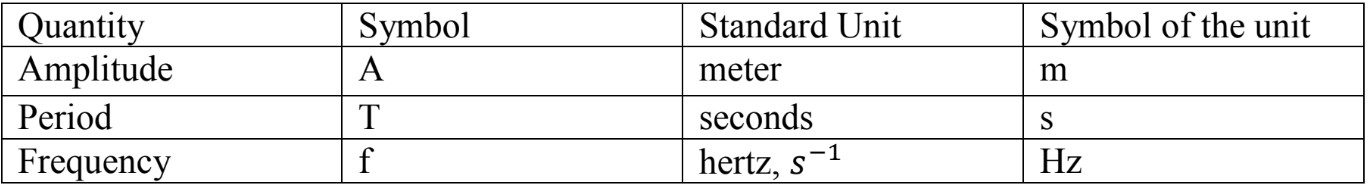• The following equations represent the mathematical relationship between frequency and period of motion;

$\large f = \frac{1}{T} \quad$ and $\quad\large T = \frac{1}{f}$

Position as a Function of Time
Since the motion is considered as a periodic motion, we would be able to plot position Vs. time graph.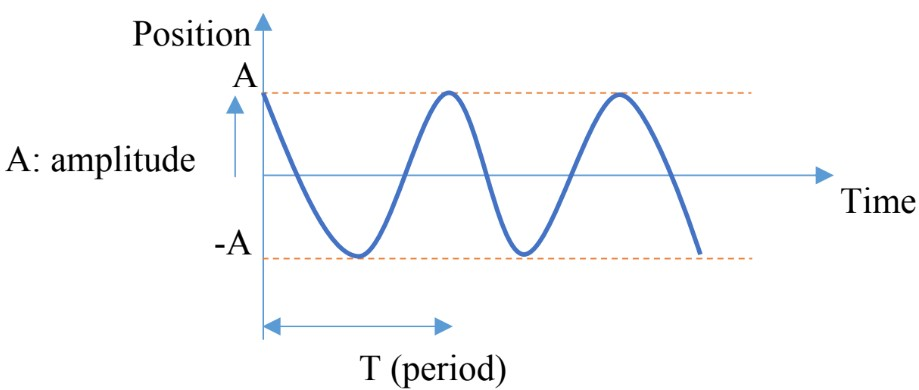Looking at the graph we can refer to it as the cosine function, since at t = 0 the position is maximum;

$x \, (t) = Acoswt$

$w =$ angular velocity
$w = 2 \pi f = 2 \pi / T$

$x \, (t) = Acos(2 \pi f t) = Acos$ $\large (\frac{2 \pi t}{T})$

• For one complete cycle,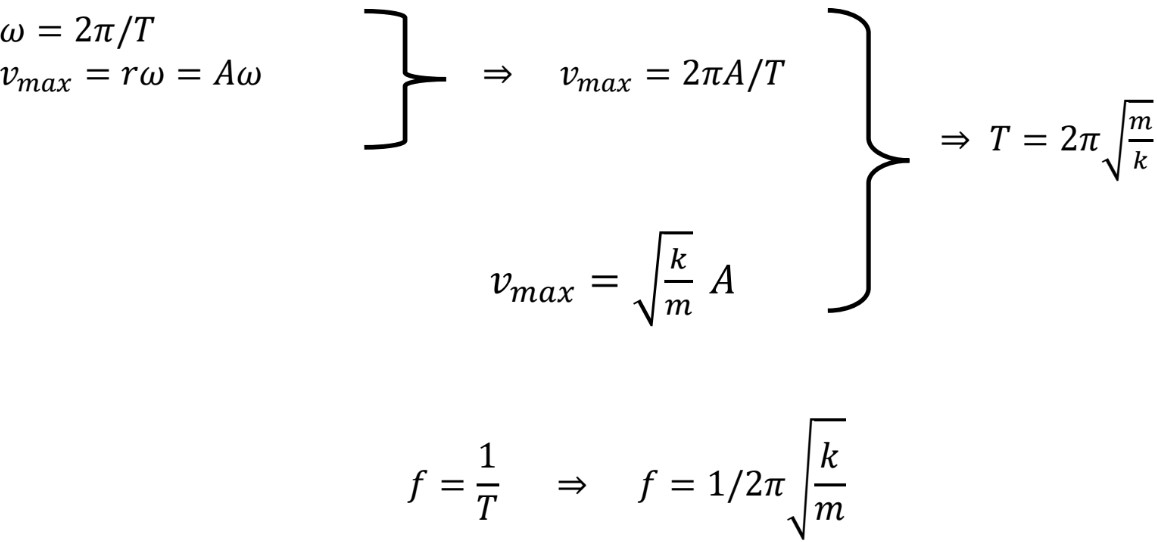Velocity as a Function of Time

• Velocity is defined as the derivative of position with respect to time,

$v =$ $\large \frac{dx}{dt}$ $=$ $\large \frac{d}{dt}$ $(Acoswt) = - Aw (sinwt)$

Since the Velocity is the sine function of time, at $t = 0, V = 0$, therefore; the graph starts at zero.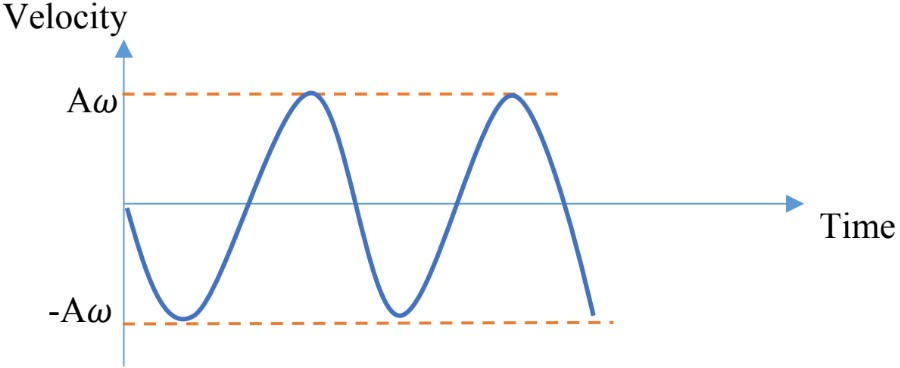Acceleration as a Function of Time

• Acceleration is defined as the derivative of velocity with respect to time,

$a =$ $\large \frac{dv}{dt}$ $=$ $\large \frac{d}{dt}$ $(-Awsinwt) = - Aw^{2} \, (coswt)$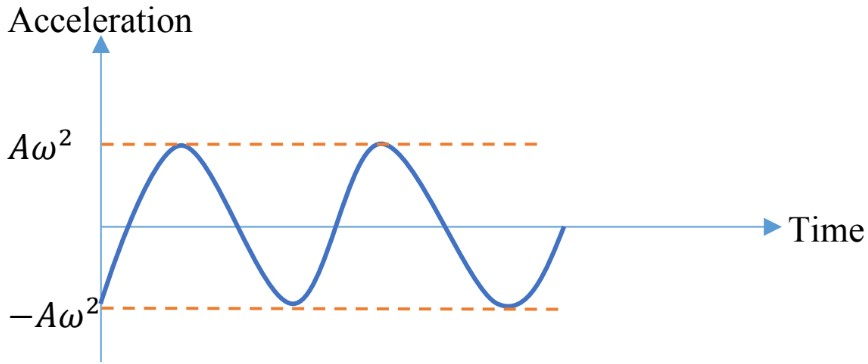$a =$ $\large \frac{F}{m}$ $= -$ $\large \frac{kx}{m}$ $=-$ $\large (\frac{kA}{m})$ $coswt = - a_{max} coswt$

$\Rightarrow a_{max} =$ $\large \frac{kA}{m}$

Simple Pendulum

• A simple pendulum is a small mass attached to the end of a string.
• The pendulum swings back and forth, ignoring the air resistance, it resembles simple harmonic motion.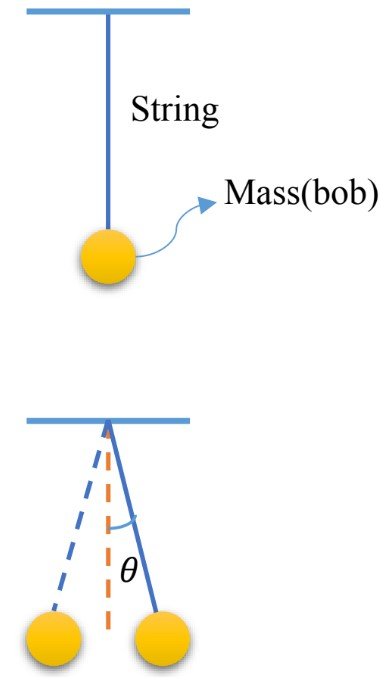Let’s apply the simple harmonic oscillator to the case of the simple pendulum;

$x(t) = Acos$$\large (\frac{2 \pi}{T} \, t)$

In the case of simple pendulum;
$A$; is the maximum angular displacement, $\theta _{max}$
$x$; is the angle the pendulum is at; the angle is measured from the equilibrium positon (the vertical position),$\theta$.

$\theta \, (t) = \theta_{max} cos$ $\large (\frac{2 \pi}{T}\, t)$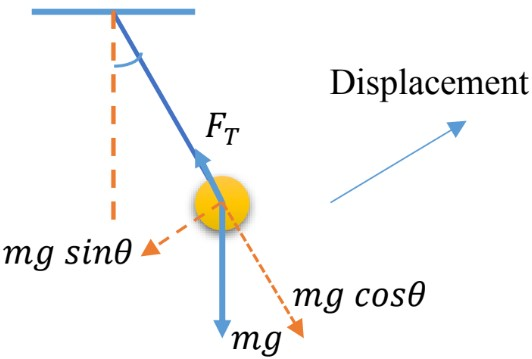• The restoring force is opposite to the displacement and is equal to the component of the weight;

• $F = - mgsin \theta$

• In this case, the motion is considered to be simple harmonic motion if the angle is less than 15°, for small angles, $\sin \theta \approx \theta$;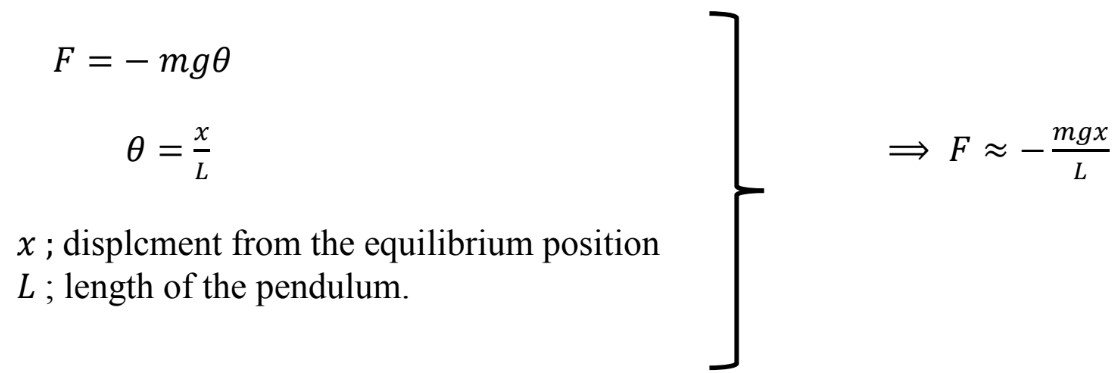Form Hooke’s law;
$F = - Kx$
$\qquad \qquad \qquad \qquad \qquad \qquad \Rightarrow K =$ $\large \frac{mg}{L}$

$F \approx -$ $\large \frac{mgx}{L}$

We know from spring- mass system;

$T = 2 \pi \, \sqrt{\frac{m}{k}} \, \Rightarrow \, T = 2 \pi \, \sqrt{\frac{m}{mg\, / \, L} } \, \Rightarrow \, T = 2 \pi \, \sqrt{\frac{L}{g}}$

and $f =$ $\large \frac{1}{2 \pi}$ $\sqrt{\frac{g}{L}} \enspace K = \frac{mg}{L}$